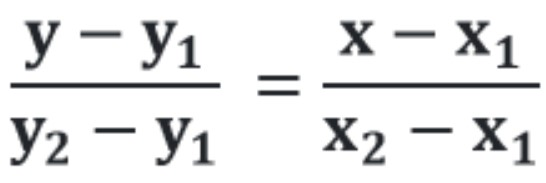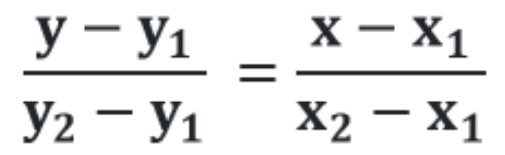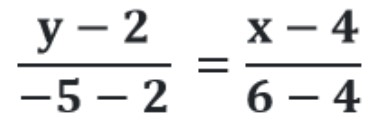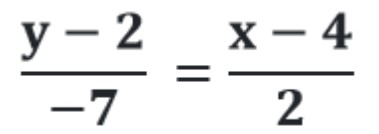# Two point Slope form calculator - Find equation of a straight line

Enter coordinates of two points in below boxes to find the equation of straight line using point slope form calculator with two points.

Give Us Feedback

Two point form calculator is an online tool that finds the equation of a line if two points are given. It calculates the point slope form equation by using 2 points of a straight line.

Equation of a straight line can also be evaluated with one point and slope using our point slope form calculator.

## Two point slope form equation

The formula to find equation of straight line with two points is:Where,

x1, x2 are points on x-axis,

y1, y2 are points on y-axis.

## Finding equation of a straight line with 2 points

Below, you can find the example of a line passing through two points.

Example:

Find the equation of line if it is passing through (4, 2) and (6, -5).

Solution:

Step 1: Write down the point slope form equation of two points.Step 2: Substitute the values in the equation.Cross multiply the above equation.

2(y - 2) = -7(x - 4)

2y – 4 = -7x + 28

7x + 2y = 32

To get the slope intercept of a line, use our slope intercept form calculator.

### Math Tools